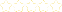• > Precalc-Chapter 9
+# Precalc-Chapter 9

##### Description:
This collection contains all lessons for Chapter 9.

### Your Quiz Points in this Playlist

Total Possible
0
8 Tutorials in this Playlist

#### Precalc Lesson 9.1 "Basic Combinatorics"

Rating:(0)

#### Precalc Lesson 9.3 "Probability" (Theoretical & Experimental)

Rating:(0)

#### Precalc lesson 9.3 "Probability" (Mutually Exclusive)

Rating:(2)

#### Precalc Lesson 9.3 "Probability" (Independent & Dependent)

Rating:(1)

#### Precalc Lesson 9.4 "Sequences" (Arithmetic Sequences)

Rating:(4)

#### Precalc Lesson 9.4 "Sequences" (Geometric Sequences)

Rating:(3)

#### Precalc Lesson 9.5 "Series" (Arithmetic Series)